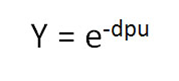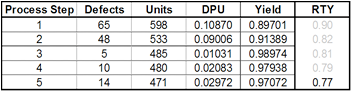# Rolled Throughput Yield

Rolled Throughput Yield (RTY) is a process performance measure that provides insight into the cumulative effects of an entire process. RTY measures the yield for each of several process steps and provides the probability that a unit will come through that process defect-free.

RTY allows us to expose the "hidden factory" by providing visibility into the yield of each process step. This helps us identify the poorest performing process steps and gives us clues into where to look to find the most impactful process improvement opportunities.

### Calculating Rolled Throughput Yield (RTY):

RTY is found by multiplying the Yields of each process step. Let's take the 5-step process below and calculate its Rolled Throughput Yield using the multiplication method mentioned above.• The calculation is: RTY = 0.90*0.91*0.99*0.98*0.97 = 0.77
• Therefore, RTY = 77%.

You may have noticed that In order to calculate RTY we must determine the Yield for each process step (which we have not covered yet). Before we get into calculating Yield, there are a few abbreviations that need to be declared.

#### Abbreviations

• Defects = D
• Unit = U
• Defects per Unit = DPU
• Yield = Y
• e = 2.71828 (mathematical constant)

### Calculating YieldThe Yield of a process step is the success rate of that step or the probability that the process step produces no defects. In order to calculate Yield we need to know DPU and then we can apply it to the Yield equation above.For example, suppose a process step has a DPU of 0.109 or 10.9% which is derived by dividing the number of defects (65) by units (598). Then, we can apply the Yield equation: Y = 2.718 ^ -0.109 = 0.8967 rounded, Y = 90%

The table above uses the above process yield data that we used in the earlier RTY calculation. This table allows us to see the DPU and Yield of each step as well as the RTY for the whole process.

### RTY: Using an Estimate of Yield

It is also possible to “estimate” Yield by taking the inverse of DPU or simply subtracting DPU from 1. Referring to the table above, let's run a quick yield estimation.

Yield Estimation = 1-DPU

• Yield Estimate for Process step 1 = 1 - 0.10870 = 0.89
• Yield Estimate for process step 2 = 1 - 0.09006 = 0.91
• Yield Estimate for process step 3 = 1 - 0.01031 = 0.99
• Yield Estimate for process step 4 = 1 – 0.02083 = 0.98
• Yield Estimate for process step 5 = 1 – 0.02972 = 0.97

RTY using the Yield Estimation Method = 0.89*0.91*0.99*0.98*0.97 = 0.76 or 76% PRETTY DARN CLOSE! And you don't even need the yield equation.